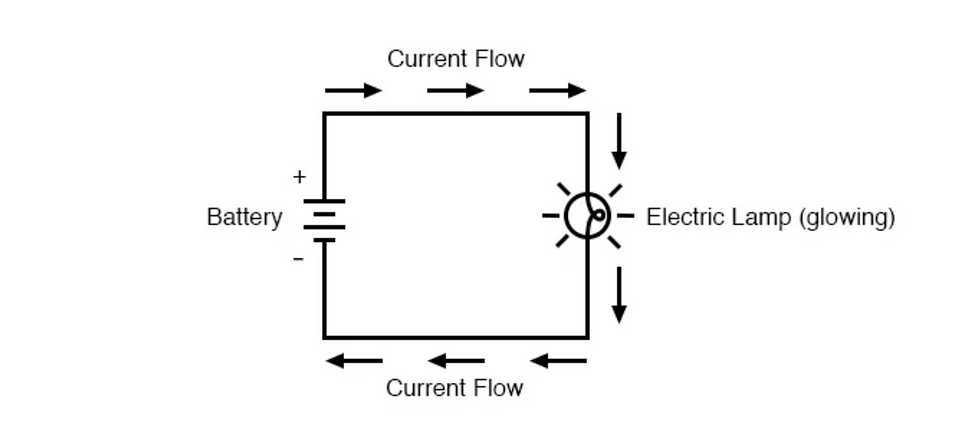top of page
Search

# Current Electricity (All about V, I, R, Q and t)Electric current is produced when electrons flow. These electrons always flow from a negatively charged (-) to a positively charged (+) .

Now coming to the convectional current, current flows from positive to negative end The diagram below makes it clearer.Electric current (I) is a measure of the rate of flow of electric charge (Q) through a given cross section of a conductor.

Such that:

I = Q / t

The SI unit of current is ampere (A). An ammeter is used to measure current.

Now before moving on, you should all be aware of how a simple circuit looks like.In the circuit shown above, it consists of:

1. a source of electromotive force that drives electric current (e.g. battery)

2. a load on which moving charges can do a useful job (e.g. a bulb)

3. conductors to connect the components together (e.g. copper wire)

4. switch to open or close a circuit.

Electromotive Force and Potential Difference:

Electromotive force (e.m.f) is the energy required to move a unit positive charge from one end of the circuit to another. Such that:

E = W / Q

where E is the e.m.f. of the power supply, W is the amount of electrical energy converted from electrical to non-electrical forms (work done) and Q is the amount of charge. The SI unit for e.m.f. is Joule per Coulomb or volt (V).

** Remember that e.m.f. is the movement of charge through the entire circuit.Remember that e.m.f. is the movement of charge through the entire circuit.The diagram above shows the voltage calculated of the cell, and as cell is providing voltage to the entire circuit, it is hence e.m.f.

Now, potential difference (p.d.) is the amount of electrical energy consumed to move a unit positive charge from one point to another in an electrical circuit. Such that:

V = W / Q

where V is the p.d., W is the electrical energy converted to other forms and Q is the amount of charge. The SI unit for this is the same as that for e.m.f. and that is volt (V).The diagram shows how p.d. can be calculated across the bulb (between two points).Resistance:

Resistance, as the name suggests, is the measure of how difficult it is for an electric current to pass through a material, copper wire let’s say. So it is basically the restriction (resistance) of a material to the free moving electrons in the material. If you compare it with the friction in moving objects, it’s quite correct.

Now in more scientific terms, resistance R of a component is the ratio of the potential difference across it to the current I flowing through it, such that:

R = V / I

where R is resistance, V is the p.d. across the component (note that across a component, that is, between two points, it’s p.d. and not e.m.f.) and I is the current flowing through it.

The SI unit of resistance is ohm (Ω).

Resistance is measured using a conductor called resistor. Resistors are of two types: fixed and variable (rheostats). Now you can tell exactly from the name what these are, right? Fixed resistors have a fixed value while variable resistors can vary the resistance and are used in circuits to vary current.

Resistivity:

Apart from temperature, there are other factors as well on which R depends. As for temperature, the higher the temperature of metallic wire, the larger the resistance.

The resistance depends on

1. the length l of the wire,

2. the cross-sectional area A or thickness of the wire, and

3. the type of material.

To memorize how these factors affect the resistivity of the conductor, memorize the following formula:

R = p (l / A)

where R is the resistance, p (a constant) is the resistivity, l is the length and A is the cross-sectional area of the wire.

** Recall the fat cat trying to go through the long / short or wide / narrow pipe. Will it be difficult or easy? If its easy means resistance is low. If it’s difficult means that resistance is high.